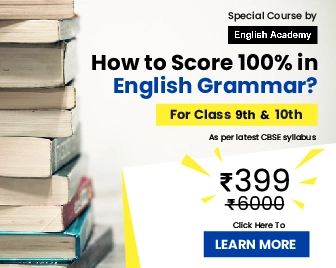Your No1 source for Latest Entrance Exams, Admission info

 Score 100 percent in English Grammar! Special Course by English Academy Experts - 55 Lessons, 40 Tests for Everyone in CBSE Class 6 to 10 Students - Check out Special Offer englishacademy.successcds.net

Home >> Class 10 >> Science >>

# Light Reflection and Refraction Important Questions 1,2,3,5 Markers CBSE Class 10 Science Chapter 10

## NCERT Solutions for Class 10 Science Chapter 10 Light Reflection and Refraction Important Questions

Here are the Light Reflection and Refraction important questions of 1,2,3 and 5 Marks for CBSE Class 10 Science Chapter 10. The important questions we have compiled will help the students to brush up on their knowledge about the subject. Students can practiceClass 10 Science important questions to understand the subject better and improve their performance in the board exam. The solutions provided here will also give students an idea about how to write the answers. Take Free Online MCQ Test for Class 10

### Light Reflection and Refraction Important Question for CBSE Class 10 Science Chapter 10

Light Reflection and Refraction Important Questions Video

 Light Reflection and Refraction MCQs 1 Mark MCQ Questions 1 Mark Important Questions 2 Mark Questions 3 Mark Questions 5 Mark Questions

### Light Reflection and Refraction Class 10 Science MCQs (1 Mark)

1. A convex mirror used for the rear view on an automobile has a focal length of 2.5m. A car is located at a distance of 4m from the mirror. In the above scenario the sign that should be assigned to f and u is

(a) u positive, f negative

(b) f positive, u negative

(c) f and u are positive

(d) f and u negative

Answer - 1) b

2. In a concave mirror an erect and virtual image is formed when the object is placed

(a) Between C and F

(b) Beyond C

(c) Between P and F

(d) At C

Answer - 2) c

3. The diameter of the reflecting surface of a spherical mirror is called its

(a) Centre of curvature

(b) R=2f

(c) Aperture

(d) Principal focus.

Answer - 3) c

4. As light travels from a rarer to a denser medium it will have

(a) increased velocity

(b) decreased velocity

(c) decreased wavelength

(d) both

Answer - (b) and (c)

5. The angle of incidence i and refraction r are equal in a transparent slab when the value of i is

(a) 0°

(b) 45°

(c) 90°

(d) depend on the material of the slab

Answer - (a) When the incident ray falls normally on the glass slab, it will refracted without deviation, i.e. along the normal in the glass slab. So, ∠i = ∠r = 0

See Light Reflection and Refraction Important Questions Video

Top

6. A divergent lens will produce

(a) always real image

(b) always virtual image

(c) both real and virtual image

(d) none of these

Answer - (b) Diverging (concave) lens will always forms a virtual, erect and diminished image.

Light Reflection and Refraction  Class 10 Science 1 Mark Important Questions

Q1. How is the refractive index of a medium related to the speed of light? Obtain an expression for refractive index of a medium with respect to another in terms of speed of light in these two media?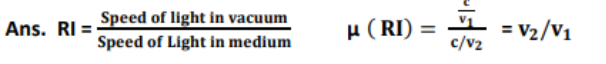Q2. Refractive index of diamond with respect to glass is 1.6 and the absolute refractive index of glass is 1.5. Find out the absolute refractive index of diamonds.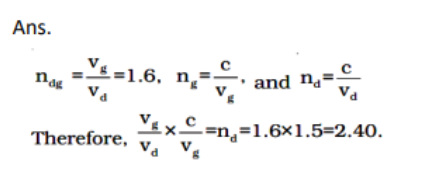Q3. Draw a ray diagram showing the path of rays of light when it enters with oblique incidence (i) from air into water; (ii) from water into air.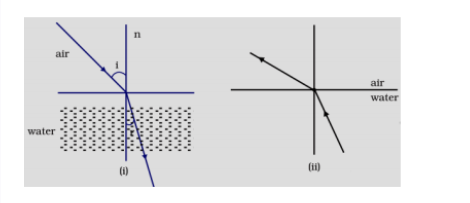### Light Reflection and Refraction Class 10 Science 2 Marks Questions

Q1. A convex lens of focal length 20 cm can produce a magnified virtual as well as real image. Is this a correct statement? If yes, where shall the object be placed in each case for obtaining these images?

Answer - Yes, it is correct. If the object is placed within 20 cm from the lens in the first case and between 20 cm and 40 cm in the second case.

Q2. Define power of a lens. What is its unit? One student uses a lens of focal length 50 cm and another of - 50 cm. What is the nature of the lens and its power used by each of them?

Answer - P = 1/f where f is in metre. Its unit is Dioptre. Lens is convex in the first case and concave in the second case. Power is equal to 2 dioptre in the first case and - 2 dioptre in the second case.
The image of a candle flame formed by a lens is obtained on a screen placed on the other side of the lens. If the image is three times the size of the flame and the distance between lens and image is 80 cm, at what distance should the candle be placed from the lens?

Q3. What is the nature of the image at a distance of 80 cm and the lens?

Answer -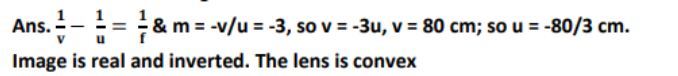Q4. Size of image of an object by a mirror having a focal length of 20 cm is observed to be reduced to 1/3rd of its size. At what distance the object has been placed from the mirror? What is the nature of the image and the mirror?

Answer  -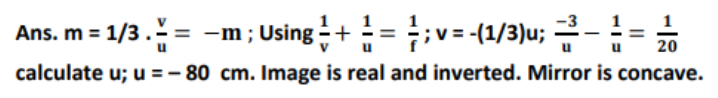### Class 10 Science Light: Reflection and Refraction 3 Marks Questions

Q1. Write laws of refraction. Explain the same with the help of ray diagram, when a ray of light passes through a rectangular glass slab.

Answer -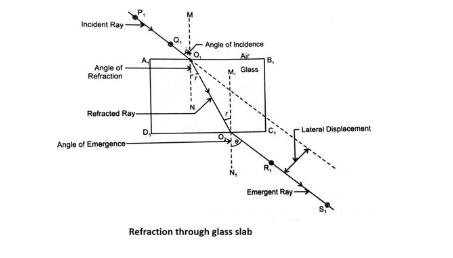Explanation: 1. Incident ray, Refracted ray and normal lie in the same plane

2. 𝑺𝒊𝒏 𝒊/ 𝑺𝒊𝒏 𝒓 = constant ( refractive index =µ)

When ray goes from rare to denser medium it bends towards the normal and when denser to rarer, it does from away from the normal. Incident ray and emergent ray are parallel to each other

Q2. Draw ray diagrams showing the image formation by a convex mirror when an object is placed (a) at infinity (b) at finite distance from the mirror.

Answer  -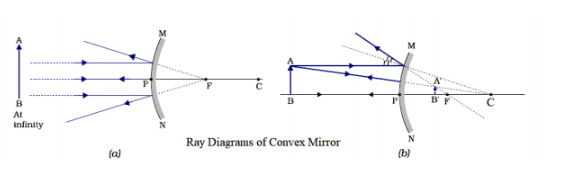Important Videos Links

### Class 10 Science Light: Reflection and Refraction MCQs (5 Marks)

Q1. A student focused the image of a candle flame on a white screen using a convex lens. He noted down the position of the candle screen and the lens as under Position of candle = 12.0 cm Position of convex lens = 50.0 cm Position of the screen = 88.0 cm

(i) What is the focal length of the convex lens?

(ii) Where will the image be formed if he shifts the candle towards the
lens at a position of 31.0 cm?

(iii) What will be the nature of the image formed if he further shifts the
candle towards the lens?

(iv) Draw a ray diagram to show the formation of the image in case (iii)
as said above.

Answer -
Draw ray diagrams showing the image formation by a concave mirror when an object is placed

(a) between pole and focus of the mirror

(b) between focus and centre of curvature of the mirror

(c) at centre of curvature of the mirror

(d) a little beyond centre of curvature of the mirror

(e) at infinity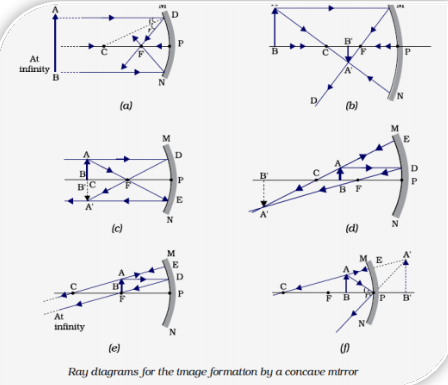Important Questions Videos Links